## The spectrum of a distant star shows that one in 2 e6 of the atoms of a particular element is in its first excited state 7.5 eV above the gr

Question

The spectrum of a distant star shows that one in 2 e6 of the atoms of a particular element is in its first excited state 7.5 eV above the ground state. What is the temperature of the star? (You can ignore the other excited states and assume the ratio of statistical weights is 4

in progress 0
6 months 2021-07-29T02:27:36+00:00 1 Answers 5 views 0

The temperature of star is 5473.87 K

Explanation:

Given:

Energy difference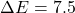eV

The ratio of number of particle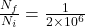Degeneracy ratio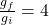From the formula of boltzmann distribution for population levels,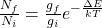Where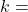boltzmann constant =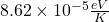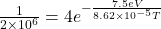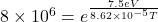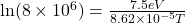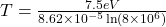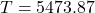K

Therefore, the temperature of star is 5473.87 K